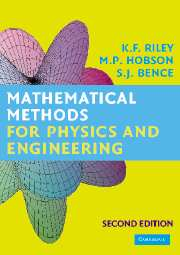## Wednesday, July 29, 2015

### Mathematical Methods for Physics and Engineering Second Edition by K. F. Riley and M. P. HobsonThe new edition of this highly acclaimed textbook contains several major additions, including more than four hundred new exercises (with hints and answers). To match the mathematical preparation of current senior college and university entrants, the authors have included a preliminary chapter covering areas such as polynomial equations, trigonometric identities, coordinate geometry, partial fractions, binomial expansions, induction, and the proof of necessary and sufficient conditions. Elsewhere, matrix decompositions, nearly-singular matrices and non-square sets of linear equations are treated in detail. The presentation of probability has been reorganized and greatly extended, and includes all physically important distributions. New topics covered in a separate statistics chapter include estimator efficiency, distributions of samples, t- and F- tests for comparing means and variances, applications of the chi-squared distribution, and maximum likelihood and least-squares fitting. In other chapters the following topics have been added: linear recurrence relations, curvature, envelopes, curve-sketching, and more refined numerical methods.